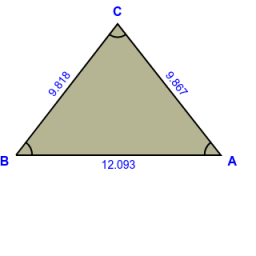Lengths of medians from coordinates

There is a triangle ABC: A [-6.6; 1.2], B [3.4; -5.6], C [2.8; 4.2]. Calculate the lengths of its medians.

t1 =  9.8843
t2 =  9.8478
t3 =  7.7666

Step-by-step explanation:

Try calculation via our triangle calculator.Did you find an error or inaccuracy? Feel free to write us. Thank you!Tips to related online calculators
Looking for help with calculating arithmetic mean?
Line slope calculator is helpful for basic calculations in analytic geometry. The coordinates of two points in the plane calculate slope, normal and parametric line equation(s), slope, directional angle, direction vector, the length of the segment, intersections of the coordinate axes, etc.
Looking for a statistical calculator?
Check out our ratio calculator.
Our vector sum calculator can add two vectors given by their magnitudes and by included angle.
Pythagorean theorem is the base for the right triangle calculator.